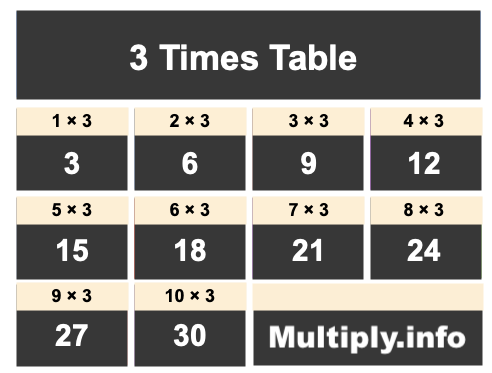3 Times TableThe picture above shows the first ten numbers in the 3 Times Table. It is created my multiplying 3 by one, then by two, then by three, and so on all the way up to ten.

Students and others use our 3 Times Table for many things, such as to find patterns or for memorizing products of numbers multiplied by 3.

In addition to the image of the 3 Times Table above, we have also written the 3 Times Table below showing 3 multiplied by every number up to one hundred. The list is in ascending order.

We created the 3 Times Table below to make it easy to read and print. We hope you will find our 3 Times Table helpful.

3 x  0 = 0
3 x  1 = 3
3 x  2 = 6
3 x  3 = 9
3 x  4 = 12
3 x  5 = 15
3 x  6 = 18
3 x  7 = 21
3 x  8 = 24
3 x  9 = 27
3 x 10 = 30
3 x 11 = 33
3 x 12 = 36
3 x 13 = 39
3 x 14 = 42
3 x 15 = 45
3 x 16 = 48
3 x 17 = 51
3 x 18 = 54
3 x 19 = 57
3 x 20 = 60
3 x 21 = 63
3 x 22 = 66
3 x 23 = 69
3 x 24 = 72
3 x 25 = 75
3 x 26 = 78
3 x 27 = 81
3 x 28 = 84
3 x 29 = 87
3 x 30 = 90
3 x 31 = 93
3 x 32 = 96
3 x 33 = 99
3 x 34 = 102
3 x 35 = 105
3 x 36 = 108
3 x 37 = 111
3 x 38 = 114
3 x 39 = 117
3 x 40 = 120
3 x 41 = 123
3 x 42 = 126
3 x 43 = 129
3 x 44 = 132
3 x 45 = 135
3 x 46 = 138
3 x 47 = 141
3 x 48 = 144
3 x 49 = 147
3 x 50 = 150
3 x 51 = 153
3 x 52 = 156
3 x 53 = 159
3 x 54 = 162
3 x 55 = 165
3 x 56 = 168
3 x 57 = 171
3 x 58 = 174
3 x 59 = 177
3 x 60 = 180
3 x 61 = 183
3 x 62 = 186
3 x 63 = 189
3 x 64 = 192
3 x 65 = 195
3 x 66 = 198
3 x 67 = 201
3 x 68 = 204
3 x 69 = 207
3 x 70 = 210
3 x 71 = 213
3 x 72 = 216
3 x 73 = 219
3 x 74 = 222
3 x 75 = 225
3 x 76 = 228
3 x 77 = 231
3 x 78 = 234
3 x 79 = 237
3 x 80 = 240
3 x 81 = 243
3 x 82 = 246
3 x 83 = 249
3 x 84 = 252
3 x 85 = 255
3 x 86 = 258
3 x 87 = 261
3 x 88 = 264
3 x 89 = 267
3 x 90 = 270
3 x 91 = 273
3 x 92 = 276
3 x 93 = 279
3 x 94 = 282
3 x 95 = 285
3 x 96 = 288
3 x 97 = 291
3 x 98 = 294
3 x 99 = 297
3 x 100 = 300

Times Table Charts
Enter another number below to see the Times Table for that number.

4 Times Table
Did you find useful patterns in our 3 Times Table that you can use for the future? Check out the next Times Table on our list.